﻿ Electric field

# Electric fieldWhen we answered the question of where the electric forces of two charged particles would be balanced, we introduced a tiny positive test charge to find the answer. After all, the electric force needs two charges by definition. Here, we will use that same concept to introduce a new quantity, the electric field.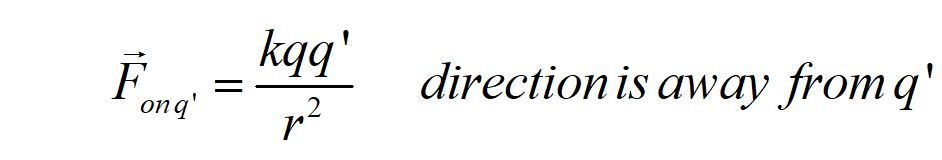We can define the electric force of charge q on charge q' and let q' be a positive tiny test charge. The direction of the force is away from the test charge.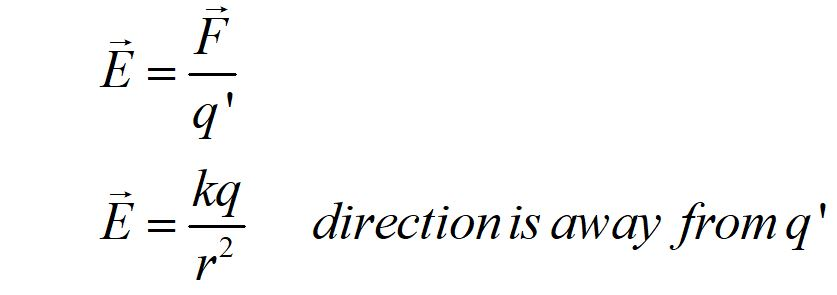If we divide through by the charge q' we can define a new quantity, the electric field. This force per charge can be defined anywhere whether there is actually a charged particle present or not. A field is constructed of a quantity that can be defined at any point. The electric field is a vector field. At every point in space, the magnitude and direction of the field can be determined.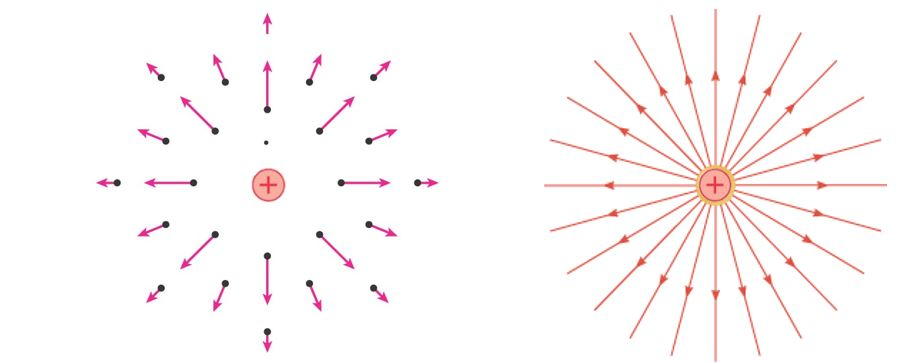The electric field of a positive point charge is shown here. We can measure the force per charge at each point in space that would exist if there was a tiny test charge there. We assume the test charge is tiny so it does not affect the field we are measuring. By convention, we use a positive test charge. The direction of the field is away from the positive test charge.

Sometimes we connect the force vector arrows to depict the field in another way, as electric field lines. The direction of the field lines has the same convention as the electric field vectors. The denser the field lines, the stronger the magnitude of the electric field.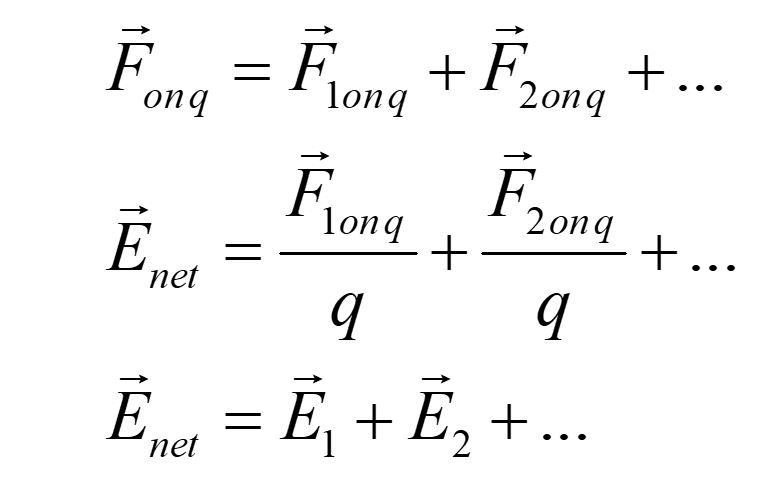The electric field is the force per charge. For a group of point charges, the E field vectors add, just like the electric force vectors.

# Sample question

## 1. What is the direction of the electric field at point P? Assume all charges are equal.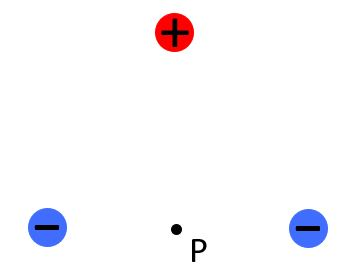# Example problem

## Find the E field of 3 equal point charges separated by a distance yi on the y-axis, at a point P, a distance r away on the x-axis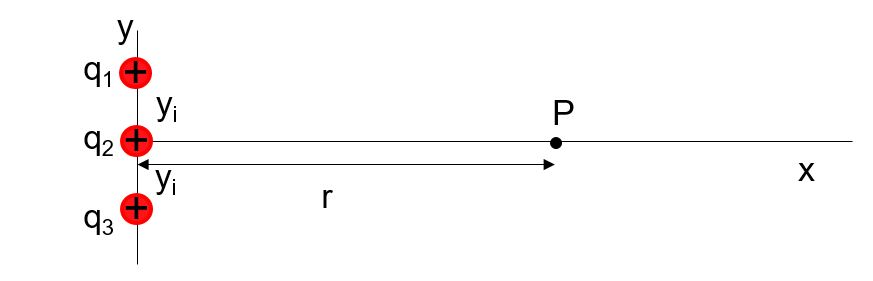### First, draw the E field vectors at the point P.### Now take advantage of the symmetry. Notice that the y components of the E field vectors from the upper and lower charges cancel. The net E field is just the contribution from E2 and twice the x-component of E1.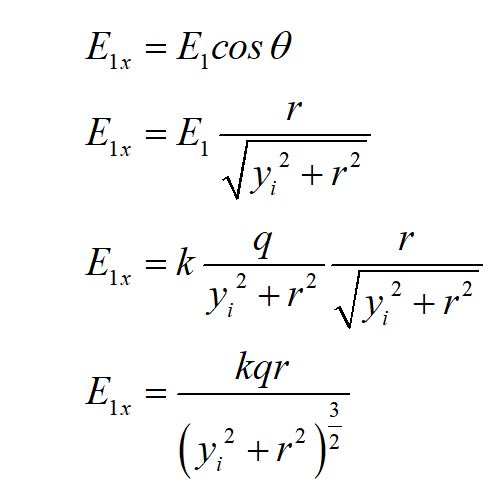### Define the x-component of E1 in terms of given variables.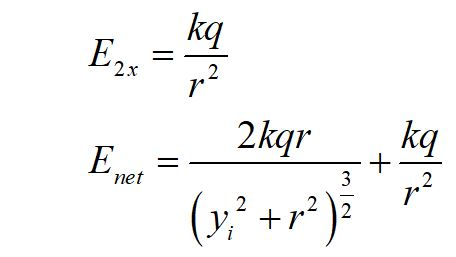## Limiting cases

### Let's check our result by looking at limiting cases. What happens when we move very close to the charges along the x-axis?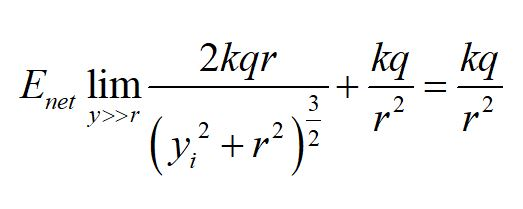### What happens if we move our point P very far away?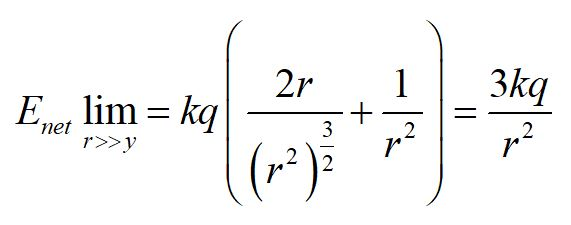### This equation is the electric field of a point charge with a charge 3q. As we get far away, the three charges appear as one, as we might expect.